# Create a Jigsaw Tile from an Existing Image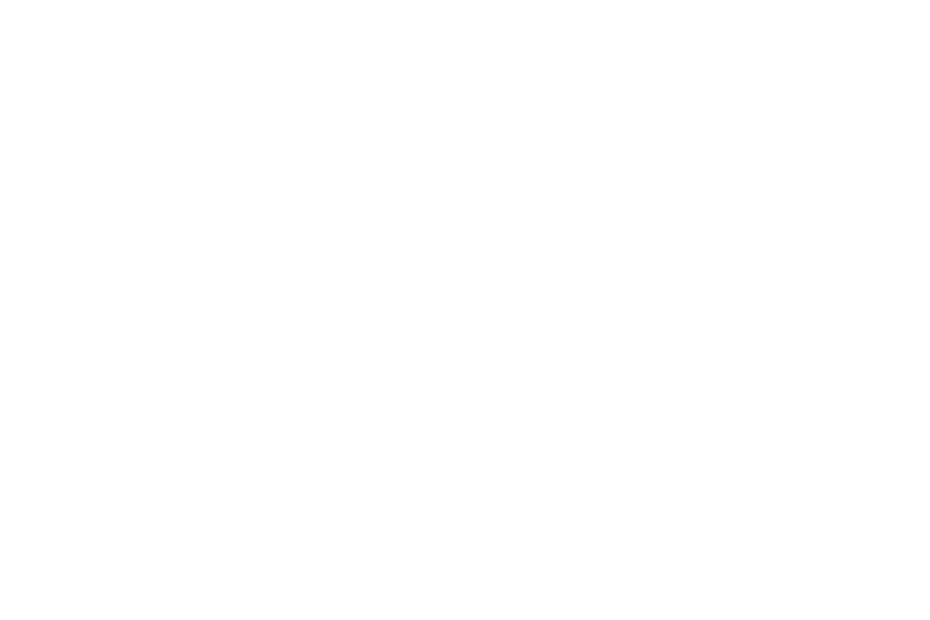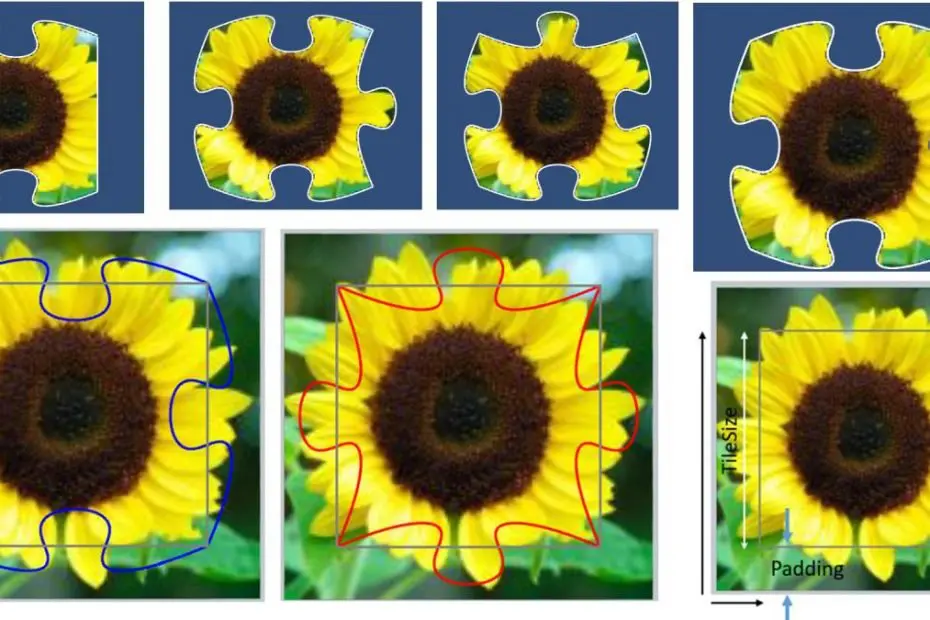This tutorial will teach how to create a Jigsaw tile from an existing image using the Bezier curve. This tutorial is the second part of the tutorial from a larger tutorial on Creating a Jigsaw Puzzle Game in Unity.

In Part 1, Implement Bezier Curve using C# in Unity, we learnt about Bezier curves and have implemented C# codes to create and display Bezier curves in Unity.

Find the GitHub repository of this tutorial at https://github.com/shamim-akhtar/jigsaw-puzzle.

Read Part 1: Implement Bezier Curve using C# in Unity
Read Part 2: Create a Jigsaw Tile from an Existing Image.
Read Part 3: Create a Jigsaw Board from an Existing Image.
Read Part 4: Create a Jigsaw Puzzle Game in Unity

Contact me:

## Part 2: Create a Jigsaw Tile from an Existing Image

Now that we know how to create a Bezier curve, we will apply that knowledge to cut an image using Bezier curves. Before we get into the details on the main topic, let’s start a scene and do some basic setup to test and display our features as we move along.

### The Scene

Please create a new scene and name it JigsawTileGen_Viz. This scene will be our sample scene to test out the various features that we will implement in the following sections.

• Add an Empty GameObject to the scene. Call the game object as TileGen.
• Select TileGen from the Hierarchy window, go to Inspector and add a New Script component. Name this script as TileGen.
• Double-click and open TileGen.cs in Visual Studio or your favourite IDE.
• Add the following code to load an image and display it as a sprite.
``````public class TilesGen : MonoBehaviour
{
public string ImageFilename;

private Texture2D mTextureOriginal;

void Start()
{
CreateBaseTexture();
}
void CreateBaseTexture()
{
// Load the main image.
{
Debug.Log("Texture is not readable");
return;
}

SpriteRenderer spriteRenderer =
spriteRenderer.sprite = SpriteUtils.CreateSpriteFromTexture2D(
mTextureOriginal,
0,
0,
mTextureOriginal.width,
mTextureOriginal.height);
}
}

```Code language: C# (cs)```
• Create a new C# file and name it SpriteUtils. In this file, we will have some utility functions for sprites.
• Add the following code to SpriteUtils.
``````public class SpriteUtils
{
public static Sprite CreateSpriteFromTexture2D(
Texture2D SpriteTexture,
int x,
int y,
int w,
int h,
float PixelsPerUnit = 1.0f,
SpriteMeshType spriteType = SpriteMeshType.Tight)
{
Sprite NewSprite = Sprite.Create(
SpriteTexture,
new Rect(x, y, w, h),
new Vector2(0, 0),
PixelsPerUnit,
0,
spriteType);
return NewSprite;
}

public static Texture2D LoadTexture(string resourcePath)
{
Texture2D tex = Resources.Load<Texture2D>(resourcePath);
return tex;
}
}

```Code language: C# (cs)```

Please create a new folder Assets/Resources and name it Images. Download the sunflower_140.jpg image and save it in the Assets/Resources/Images folder.

Select this image and modify the settings as shown below.

Go to Unity Editor, select TilesGen and set the Image Filename field to Images/sunflower_140, as shown below.

Select the Main Camera game object from the Hierarchy and set the following values in the Inspector.

Click Play. You should see as shown below.

### The Template Bezier Curve

Before we go into the details of cutting the image, let’s define some critical foundational constraints under which our game will operate.

We will use a template Bezier curve with a set of 16 control points. These are:

``````public static readonly List<Vector2> ControlPoints = new List<Vector2>()
{
new Vector2(0, 0),
new Vector2(35, 15),
new Vector2(47, 13),
new Vector2(45, 5),
new Vector2(48, 0),
new Vector2(25, -5),
new Vector2(15, -18),
new Vector2(36, -20),
new Vector2(64, -20),
new Vector2(85, -18),
new Vector2(75, -5),
new Vector2(52, 0),
new Vector2(55, 5),
new Vector2(53, 13),
new Vector2(65, 15),
new Vector2(100, 0)
};

```Code language: C# (cs)```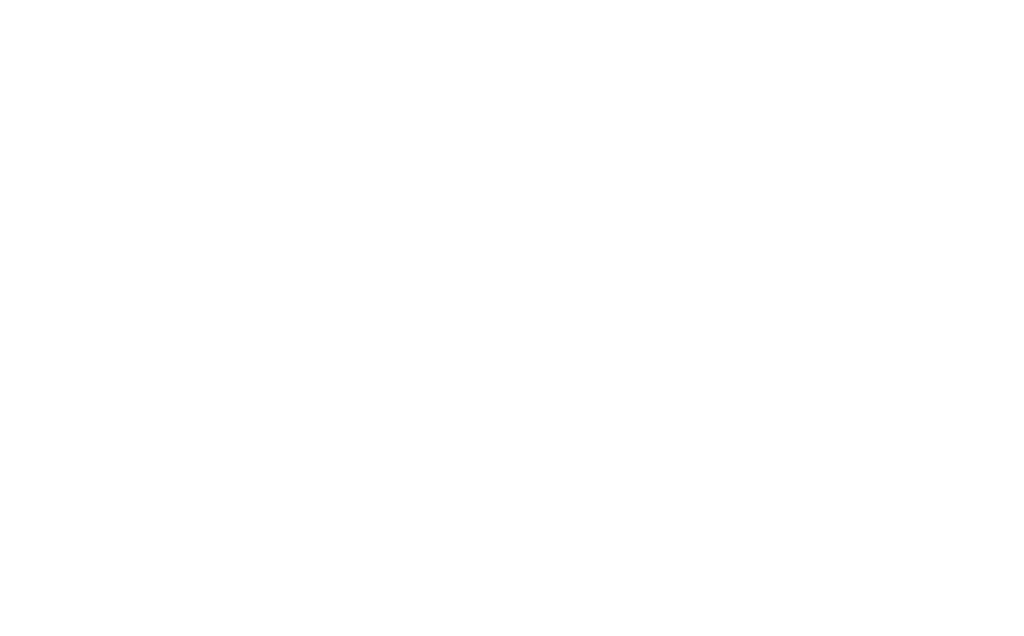The Template Bezier Curve for Jigsaw

Of course, you can experiment and create your own sets of control points that define your desired Bezier curve. These control points are for x from 0 to 100 and y from -20 to 20. The picture above shows these control points and the Bezier curve formed by these points. For the rest of this tutorial, we are going to use the above template Bezier curve.

### The Jigsaw Tile

We will standardize our Jigsaw tile to be of a regular square size of 140×140 pixels. The picture above shows one typical block of an image representing one Jigsaw tile area, with ABCD representing the square of 100×100 pixels. Next, we will apply our Bezier curves to the four sides AB, BC, CD and DA. Also, remember that we will have to use our curves in two ways. One will be the usual way, and the other will be the reflected control points along the line, as shown below.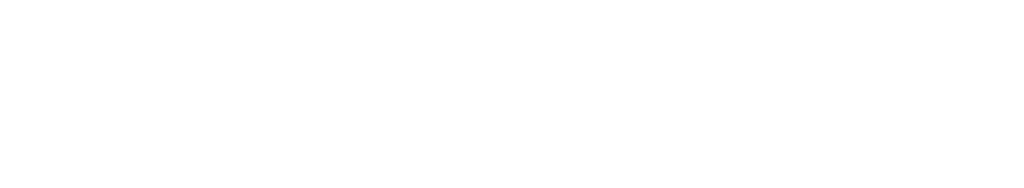The Bezier curves for creating Jigsaw tiles

To get the second Bezier curve, we simple negate the Y coordinate values.

For corner tiles, we won’t need to apply the curve. That makes it three conditions for each side. Let’s represent all these by two enumeration types.

``````// The 4 directions
public enum Direction
{
UP,
RIGHT,
DOWN,
LEFT,
}

// The operations on each of the four directions.
public enum PosNegType
{
POS,
NEG,
NONE,
}

```Code language: C# (cs)```

The picture below shows all these eight curves, with blue being the standard curve (POS) and red being the reflected curve (NEG). The straight lines in grey will represent NONE.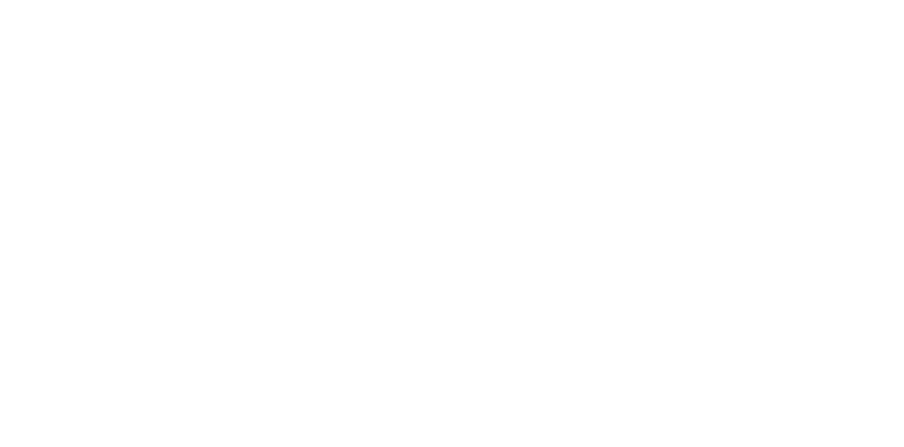The Bezier curves creating a jigsaw tile

Note that we do not have to recreate the Bezier curves again and again to achieve the above. Instead, we only have to calculate the Bezier curve once.

Then we need to transform these points to get all the other points required for the 8 Bezier curves. For curves along the vertical line, we will swap the x and y coordinates.

We are ready to manipulate the sprite with this information and data and cut (set transparency) the regions that fall exterior to the curves (or straight lines).

Create a new class called Tile in a namespace named Puzzle.

``````namespace Puzzle
{
public class Tile
{
}
}

```Code language: C# (cs)```

This class will represent our Jigsaw tile. We will add some static member variables as these variables will be common for all tiles.

``````#region Static Variables and Functions
// The padding in pixels for the jigsaw tile.
// As explained above in the diagram, we are
// having each tile of 140 by 140 pixels with
// 20 pixels padding.
public static int Padding = 20;

// The actual size of the tile (minus the padding).
public static int TileSize = 100;

// These are the control points for our Bezier curve.
// These control points do not change and are marked readonly.
public static readonly List<Vector2> ControlPoints = new List<Vector2>()
{
new Vector2(0, 0),
new Vector2(35, 15),
new Vector2(47, 13),
new Vector2(45, 5),
new Vector2(48, 0),
new Vector2(25, -5),
new Vector2(15, -18),
new Vector2(36, -20),
new Vector2(64, -20),
new Vector2(85, -18),
new Vector2(75, -5),
new Vector2(52, 0),
new Vector2(55, 5),
new Vector2(53, 13),
new Vector2(65, 15),
new Vector2(100, 0)
};

// The template Bezier curve.
public static List<Vector2> BezCurve = BezierCurve.PointList2(ControlPoints, 0.001f);
```Code language: C# (cs)```

Refer to the comments within the code. The variables are self-explanatory. We then add the enumeration types for the directions and the operations on those directions.

``````#region Enumerations
// The 4 directions
public enum Direction
{
UP,
RIGHT,
DOWN,
LEFT,
}

// The operations on each of the four directions.
public enum PosNegType
{
POS,
NEG,
NONE,
}
#endregion

```Code language: C# (cs)```

We will have a variable that will store the operation for each of the four different sides.

``````// The array of PosNegType operations to be performed on
// each of the four directions.
private PosNegType[] mPosNeg = new PosNegType
{
PosNegType.NONE,
PosNegType.NONE,
PosNegType.NONE,
PosNegType.NONE
};

```Code language: C# (cs)```

### The Modus Operandi

Before we start going into the details of our implementation, let’s look at the high-level steps to achieve the solution. First, to cut our texture based on the lines and curves, we will take the following steps:

1. Set the original texture to the tile
2. Create a new texture of the same width and height as the original texture and fill it up with complete transparency.
3. Set the newly created texture boundary based on the curves and straight lines depending on what PosNegType/s for each direction.
4. Do flood fill of the region inside this boundary. The flood fill will set the colour of the newly created texture based on the colour from the original image.

We proceed with implementing the Tile class by adding a few more variables:

``````#region Properties
public Texture2D FinalCut { get; private set; }
#endregion```Code language: PHP (php)```

FinalCut property lets the user get access to the final texture. Note that we only provide get access to this property. That means we cannot modify the FinalCut texture outside of this class.

``````// The original texture used to create this Jigsaw tile.
// We are not going to change this original texture.
// Instead, we are going to create a new texture and
// set the values to either pixels from the original
// texture or transparent (if the pixel falls outside
// the curves or straight lines (defined by the enum
// PosNegType.
private Texture2D mOriginalTex;
```Code language: PHP (php)```

mOriginalTex is a private variable that stores the input texture. We won’t modify this texture. Instead, we will create a new texture, FinalCut, and alter this new texture.

``````// The array of PosNegType operations to be performed on
// each of the four directions.
private PosNegType[] mPosNeg = new PosNegType
{
PosNegType.NONE,
PosNegType.NONE,
PosNegType.NONE,
PosNegType.NONE
};

```Code language: C# (cs)```

mPosNeg is a private variable that will store the type of operation that we will perform for a given direction. By default, the operation for all the directions is set to NONE.

We create a new public function that will let the user set the specific value of the operation for a given direction.

``````public void SetPosNegType(Direction dir, PosNegType type)
{
mPosNeg[(int)dir] = type;
}
```Code language: C# (cs)```

### The Constructor

We will now implement the constructor of this Tile class.

``````// The constructor.
public Tile(Texture2D tex)
{
int tileSizeWithPadding = 2 * Padding + TileSize;
if (tex.width != tileSizeWithPadding ||
{
Debug.Log("Unsupported texture dimension for Jigsaw tile");
return;
}

mOriginalTex = tex;

// Create a new texture with width and height as Padding + TileSize + Padding.
FinalCut = new Texture2D(
TextureFormat.ARGB32,
false);

// Initialize the newly create texture with transparent colour.
for (int i = 0; i < tileSizeWithPadding; ++i)
{
for (int j = 0; j < tileSizeWithPadding; ++j)
{
FinalCut.SetPixel(i, j, TransparentColor);
}
}
}
```Code language: C# (cs)```

### The Apply Method

The Apply method is simple. In this method, we first initialize the flood fill and then call the FloodFill function. Then, finally, we call the Apply method of the Texture2D. We need to call this method to apply the changes to the Texture2D before Unity can display the changes made to the texture pixels.

``````public void Apply()
{
FloodFillInit();
FloodFill();
FinalCut.Apply();
}

```Code language: C# (cs)```

The majority of the work happens in the FloodFill method.

So, before we implement the FloodFill method, let’s dive into what is flood fill and how to implement one.

### Flood Fill

Flood fill is an algorithm that determines and alters the area connected to a given node in a multi-dimensional array with some matching attribute. – Wikipedia.

Let’s analyze a few crucial phrases from the above definition.

Flood fill is an algorithm that determines and alters the area connected to a given node in a multi-dimensional array with some matching attribute.

For our case, “determines and alters the area” will refer to the area that falls inside the curves in all four directions.

“given node” refers to a pixel.

multi-dimensional array” refers to the two-dimensional array of pixels.

matching attribute “refers to the condition of whether or not a pixel falls in the set of points defined by the curves and straight lines.

To implement flood fill, we will follow the following algorithm:

• Step 1: Set up the boundary of the texture based on the curves and straight lines. Mark all pixels that fall in this set of points as visited.
• Step 2: Take the centre pixel of the FinalCut texture and set the colour value from the input texture’s centre pixel. Mark it as visited. Add this pixel to the stack.
• Step 3: While the stack of pixels is not empty, go up, left, right and down to get the next pixel. If the next pixel is already marked as visited, then we do not process that pixel. If not, then we set that pixel as visited, set the colour from the original texture of the same pixel, and add it to the stack.

We will make a method named FloodFillInit to perform Step 1 and Step 2 and another method named FloodFill to perform Step 3.

Before we start implementing these two methods, we will need to declare the following variables.

``````// A 2d boolean array that stores whether a particular
// pixel is visited. We need this array for the flood fill.
private bool[,] mVisited;
// A stack needed for the flood fill of the textures.
private Stack<Vector2Int> mStack = new Stack<Vector2Int>();```Code language: PHP (php)```

### FloodFillInit Method

In this method, we first initialize the mVisited Boolean array and set all the values to false. That means by default; all the pixels are not visited.

``````    int tileSizeWithPadding = 2 * Padding + TileSize;

for (int i = 0; i < tileSizeWithPadding; ++i)
{
for (int j = 0; j < tileSizeWithPadding; ++j)
{
mVisited[i, j] = false;
}
}
```Code language: C# (cs)```

Then we create the list of points defined by the Bezier curves and straight lines depending on what operation is set for each of the four directions.

``````List<Vector2> pts = new List<Vector2>();
for (int i = 0; i < mPosNeg.Length; ++i)
{
}
```Code language: C# (cs)```

Once we have the list of the points, we make all pixels that fall in this point list as mVisited to be true.

``````// Now we should have a closed curve.
// To verify check by drawing the pts to a line renderer.
for (int i = 0; i < pts.Count; ++i)
{
mVisited[(int)pts[i].x, (int)pts[i].y] = true;
}
```Code language: C# (cs)```

Finally, we take the centre pixel, mark it as visited and then push it to the stack.

``````// start from center.
Vector2Int start = new Vector2Int(tileSizeWithPadding / 2, tileSizeWithPadding / 2);

mVisited[start.x, start.y] = true;
mStack.Push(start);
```Code language: C# (cs)```

This completes our FloodFillInit function. Next, of course, we will need to implement the CreateCurve method. We will do that in the section below FloodFill.

### FloodFill Method

The flood fill method is simple. While there are more pixels to be checked, we continue getting the neighbour pixel and check if it is visited. If so, then ignore that pixel. If not, then mark that pixel as visited and push it to the stack.

``````public void FloodFill()
{
int width_height = Padding * 2 + TileSize;
while (mStack.Count > 0)
{
//FloodFillStep();
Vector2Int v = mStack.Pop();

int xx = v.x;
int yy = v.y;
Fill(v.x, v.y);

// check right.
int x = xx + 1;
int y = yy;
if (x < width_height)
{
Color c = FinalCut.GetPixel(x, y);
if (!mVisited[x, y])
{
mVisited[x, y] = true;
mStack.Push(new Vector2Int(x, y));
}
}

// check left.
x = xx - 1;
y = yy;
if (x >= 0)
{
Color c = FinalCut.GetPixel(x, y);
if (!mVisited[x, y])
{
mVisited[x, y] = true;
mStack.Push(new Vector2Int(x, y));
}
}

// check up.
x = xx;
y = yy + 1;
if (y < width_height)
{
Color c = FinalCut.GetPixel(x, y);
if (!mVisited[x, y])
{
mVisited[x, y] = true;
mStack.Push(new Vector2Int(x, y));
}
}

// check down.
x = xx;
y = yy - 1;
if (y >= 0)
{
Color c = FinalCut.GetPixel(x, y);
if (!mVisited[x, y])
{
mVisited[x, y] = true;
mStack.Push(new Vector2Int(x, y));
}
}
}
}
```Code language: C# (cs)```

Fill function sets the actual pixel colour value from the original texture to the FinalCut texture, as shown below.

``````void Fill(int x, int y)
{
Color c = mOriginalTex.GetPixel(x, y);
c.a = 1.0f;
FinalCut.SetPixel(x, y, c);
}
```Code language: C# (cs)```

### CreateCurve Method

This method creates a set of points that mark the texture’s boundary based on either the Bezier curve or the straight line. The input to this function is the direction and the operation for that direction.

``public static List<Vector2> CreateCurve(Tile.Direction dir, PosNegType type)`Code language: PHP (php)`

#### UP Direction

Let’s look at the UP direction first. There can be three types of operations that we can perform for the UP direction. They are POS, NEG or NONE. Note that the point stored in the BezCurve variable starts with x = 0 and ends at x = 100. The y fluctuates between -20 and 20. So to apply our curve in the UP direction, we will have to add an offset of Padding to x and Padding + TileSize to y.

To get the list of points for a POS operation in the UP direction, we will translate the points by the offset. So let’s write a TranslatePoints function that takes in a list of points and translates these points by an offset.

``````static void TranslatePoints(List<Vector2> iList, Vector2 offset)
{
for (int i = 0; i < iList.Count; ++i)
{
iList[i] += offset;
}
}
```Code language: C# (cs)```

To get the list of points for a NEG operation in the UP direction, we will have to invert the y values and then translate the points by the offset. So let’s write an InvertY function that takes in a list of points and negates the y values.

``````static void InvertY(List<Vector2> iList)
{
for (int i = 0; i < iList.Count; ++i)
{
iList[i] = new Vector2(iList[i].x, -iList[i].y);
}
}
```Code language: C# (cs)```

To get the list of points for a NONE operation in the UP direction, we need to accumulate the x and y values for a constant y = Padding + TileSize and x from 0 to Padding + TileSize.

The complete code for UP direction is as below.

``````public static List<Vector2> CreateCurve(Tile.Direction dir, PosNegType type)
{
int sw = TileSize;
int sh = TileSize;

List<Vector2> pts = new List<Vector2>(Tile.BezCurve);
switch (dir)
{
case Tile.Direction.UP:
if (type == PosNegType.POS)
{
TranslatePoints(pts, new Vector3(padding_x, padding_y + sh, 0));
}
else if (type == PosNegType.NEG)
{
InvertY(pts);
TranslatePoints(pts, new Vector3(padding_x, padding_y + sh, 0));
}
else if (type == PosNegType.NONE)
{
pts.Clear();
for (int i = 0; i < 100; ++i)
{
}
}
break;
}
return pts;
}
```Code language: C# (cs)```

#### Helper Functions to Visualize Curves

Before proceeding with the rest of the implementation, let’s test out the CreateCurve function for the UP direction and the various operations. To test whether our curves are correct, let’s create some helper functions to help us visualize the curves.

Our first method will be a static method to create a default LineRenderer.

``````public static LineRenderer CreateLineRenderer(Color color, float lineWidth = 1.0f)
{
GameObject obj = new GameObject();

LineRenderer lr = obj.AddComponent<LineRenderer>();

lr.startColor = color;
lr.endColor = color;
lr.startWidth = lineWidth;
lr.endWidth = lineWidth;
lr.material = new Material(Shader.Find("Sprites/Default"));
return lr;
}
```Code language: C# (cs)```

Then we will add a new private member variable.

``````private Dictionary<(Direction, PosNegType), LineRenderer> mLineRenderers =
new Dictionary<(Direction, PosNegType), LineRenderer>();
```Code language: HTML, XML (xml)```

This is to keep track of all the possible line renderers for this tile using a Dictionary.

Then we add a helper method called DrawCurve that renders the curve created by the CreateCurve method.

``````// An utility/helper function to show the curve
// using a LineRenderer.
public void DrawCurve(Direction dir, PosNegType type, Color color)
{
if (!mLineRenderers.ContainsKey((dir, type)))
{
}

LineRenderer lr = mLineRenderers[(dir, type)];
lr.startColor = color;
lr.endColor = color;
lr.gameObject.name = "LineRenderer_" + dir.ToString() + "_" + type.ToString();
List<Vector2> pts = Tile.CreateCurve(dir, type);

lr.positionCount = pts.Count;
for (int i = 0; i < pts.Count; ++i)
{
lr.SetPosition(i, pts[i]);
}
}
```Code language: C# (cs)```

We can now test and see if our curve for UP and the various operations are correct. To do so, open the TileGen.cs file. Add the following method:

``````void TestTileCurves()
{
Tile tile = new Tile(mTextureOriginal);
tile.DrawCurve(Tile.Direction.UP, Tile.PosNegType.POS, Color.red);
}```Code language: JavaScript (javascript)```

And amend the Start function as

``````void Start()
{
CreateBaseTexture();
TestTileCurves();
}```Code language: JavaScript (javascript)```

Click Play. You should see the following picture.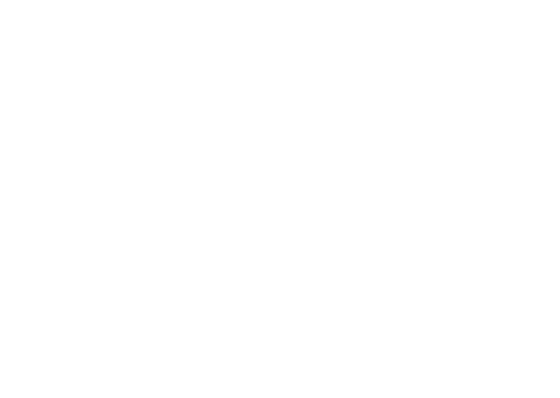Individual Bezier curve on the upper side of the image

Test out for the other two operations, NEG and NONE as well.

Modify the TestTileCurves function by adding the two curves as well.

``````void TestTileCurves()
{
Tile tile = new Tile(mTextureOriginal);
tile.DrawCurve(Tile.Direction.UP, Tile.PosNegType.POS, Color.red);
tile.DrawCurve(Tile.Direction.UP, Tile.PosNegType.NEG, Color.green);
tile.DrawCurve(Tile.Direction.UP, Tile.PosNegType.NONE, Color.white);
}
```Code language: C# (cs)```

Click Play. You should see the following picture.

We will now continue with the CreateCurve method and complete the other three directions.

#### RIGHT Direction

To get the list of points for a POS operation in the RIGHT direction, we will have to swap x and y and then translate the points by an offset of (Padding + TileSize, Padding).

So let’s write a SwapXY function that takes in a list of points and swaps the x and y values of these points.

``````static void SwapXY(List<Vector2> iList)
{
for (int i = 0; i < iList.Count; ++i)
{
iList[i] = new Vector2(iList[i].y, iList[i].x);
}
}
```Code language: C# (cs)```

We continue our implementation of the CreateCurve function from where we left in the UP section. We now implement for RIGHT.

#### RIGHT Direction

``````case Tile.Direction.RIGHT:
if (type == PosNegType.POS)
{
SwapXY(pts);
TranslatePoints(pts, new Vector3(padding_x + sw, padding_y, 0));
}
else if (type == PosNegType.NEG)
{
InvertY(pts);
SwapXY(pts);
TranslatePoints(pts, new Vector3(padding_x + sw, padding_y, 0));
}
else if (type == PosNegType.NONE)
{
pts.Clear();
for (int i = 0; i < 100; ++i)
{
}
}
break;
```Code language: C# (cs)```

#### DOWN Direction

``````case Tile.Direction.DOWN:
if (type == PosNegType.POS)
{
InvertY(pts);
}
else if (type == PosNegType.NEG)
{
}
else if (type == PosNegType.NONE)
{
pts.Clear();
for (int i = 0; i < 100; ++i)
{
}
}
break;
```Code language: C# (cs)```

#### LEFT Direction

``````case Tile.Direction.LEFT:
if (type == PosNegType.POS)
{
InvertY(pts);
SwapXY(pts);
}
else if (type == PosNegType.NEG)
{
SwapXY(pts);
}
else if (type == PosNegType.NONE)
{
pts.Clear();
for (int i = 0; i < 100; ++i)
{
}
}
break;
```Code language: C# (cs)```

Now, we test the curves for all four directions.

``````void TestTileCurves()
{
Tile tile = new Tile(mTextureOriginal);
tile.DrawCurve(Tile.Direction.UP, Tile.PosNegType.POS, Color.red);
tile.DrawCurve(Tile.Direction.UP, Tile.PosNegType.NEG, Color.green);
tile.DrawCurve(Tile.Direction.UP, Tile.PosNegType.NONE, Color.white);

tile.DrawCurve(Tile.Direction.RIGHT, Tile.PosNegType.POS, Color.red);
tile.DrawCurve(Tile.Direction.RIGHT, Tile.PosNegType.NEG, Color.green);
tile.DrawCurve(Tile.Direction.RIGHT, Tile.PosNegType.NONE, Color.white);

tile.DrawCurve(Tile.Direction.DOWN, Tile.PosNegType.POS, Color.red);
tile.DrawCurve(Tile.Direction.DOWN, Tile.PosNegType.NEG, Color.green);
tile.DrawCurve(Tile.Direction.DOWN, Tile.PosNegType.NONE, Color.white);

tile.DrawCurve(Tile.Direction.LEFT, Tile.PosNegType.POS, Color.red);
tile.DrawCurve(Tile.Direction.LEFT, Tile.PosNegType.NEG, Color.green);
tile.DrawCurve(Tile.Direction.LEFT, Tile.PosNegType.NONE, Color.white);
}
```Code language: C# (cs)```

Click Play. You should see the below picture.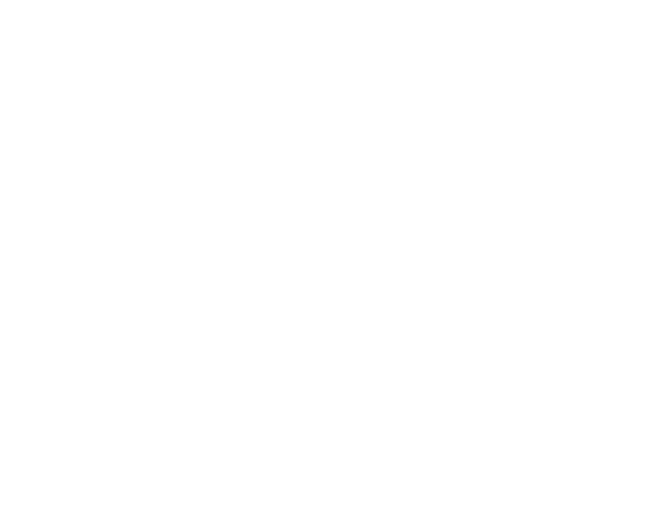Bezier curves applied to all sides of the image to create a Jigsaw tile

We have successfully tested our curves. Now, let’s see if our flood fill works. To do so, we will add the following method in TilesGen.cs.

``````void TestTileFloodFill()
{
Tile tile = new Tile(mTextureOriginal);

tile.SetPosNegType(Tile.Direction.UP, Tile.PosNegType.POS);
tile.SetPosNegType(Tile.Direction.RIGHT, Tile.PosNegType.POS);
tile.SetPosNegType(Tile.Direction.DOWN, Tile.PosNegType.POS);
tile.SetPosNegType(Tile.Direction.LEFT, Tile.PosNegType.POS);

// Uncomment the following 4 lines of code if you want to see the
// curves drawn on the tile too.
tile.DrawCurve(Tile.Direction.UP, Tile.PosNegType.POS, Color.white);
tile.DrawCurve(Tile.Direction.RIGHT, Tile.PosNegType.POS, Color.white);
tile.DrawCurve(Tile.Direction.DOWN, Tile.PosNegType.POS, Color.white);
tile.DrawCurve(Tile.Direction.LEFT, Tile.PosNegType.POS, Color.white);

tile.Apply();

SpriteRenderer spriteRenderer = GetComponent<SpriteRenderer>();

Sprite sprite = SpriteUtils.CreateSpriteFromTexture2D(
tile.FinalCut,
0,
0,
tile.FinalCut.width,
tile.FinalCut.height);
spriteRenderer.sprite = sprite;
}

```Code language: C# (cs)```

Call the above method from the Start.

``````void Start()
{
CreateBaseTexture();
//TestTileCurves(); comment this off. We do not want to see the curves.
TestTileFloodFill();
}

```Code language: C# (cs)```

Click Play. You should see the below.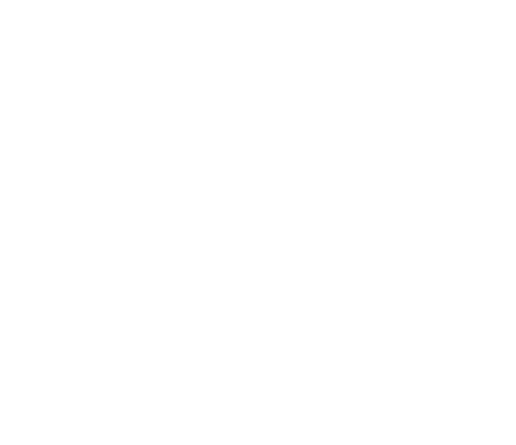A jigsaw tile from an image

Some other possible configurations are:

We have completed Part 2 of the tutorial. In the next section, Part 3 – Create a Jigsaw Board from an Existing Image, we will build the Jigsaw tileset from an image to create a Jigsaw board.

## 3 thoughts on “Create a Jigsaw Tile from an Existing Image”

1.Hello!
Thanks for the wonderful tutorial, there are not too much people nowadays on internet could write like this. I was enlightened by this.

I only have one question. It is about the Anti-Aliasing. Like the final tile image, there are many aliasings between the tile side and the white line.

I tried a simple way, to set the pixel color 0.5(or 0.3)alpha that in the ‘pts’ list。 like:
————————————————————————————————
private float[,] mVisited;

for (int i = 0; i < pts.Count; ++i)
{
mVisited[(int)pts[i].x, (int)pts[i].y] = 0.5f;
}

public void GetNetFillPoint(int x, int y)
{
//Color c = FinalCut.GetPixel(x, y);
if (mVisited[x, y] == 0)
{
mVisited[x, y] = 1;
mStack.Push(new Vector2Int(x, y));
}
else if (mVisited[x, y] != 1)
{
Fill(x, y, mVisited[x, y]);
}
}

void Fill(int x, int y, float alpha)
{
Color c = mOriginalTex.GetPixel(x + xIndex * TileSize, y + yIndex * TileSize);
c.a = alpha;
FinalCut.SetPixel(x, y, c);
}
————————————————————————————————
Produced very little effect.

Do you have any idea to anti-aliasing?

Look forward to receiving your reply, and thanks again!

1.In this game, We have the points of the tile side. It’s pretty suited to FXAA.

2.Dear Tony,

Thank you for your good words on the tutorial. Yes, I see that aliasing is an issue, and I agree that something needs to be done on this side. My tutorial is primarily on the theoretical approach to solving the problem of generating Jigsaw tiles based on the Bezier curve.

I will look into the aliasing as an issue and see how we could implement some sort of programmatically generated antialising. Let me look into in and revert.

Thanks, Shamim